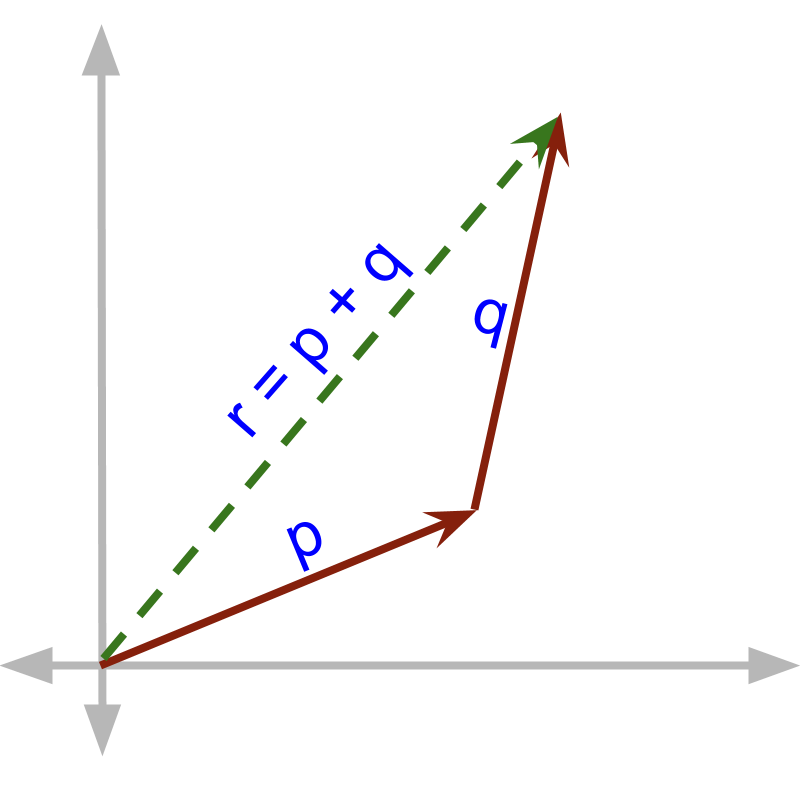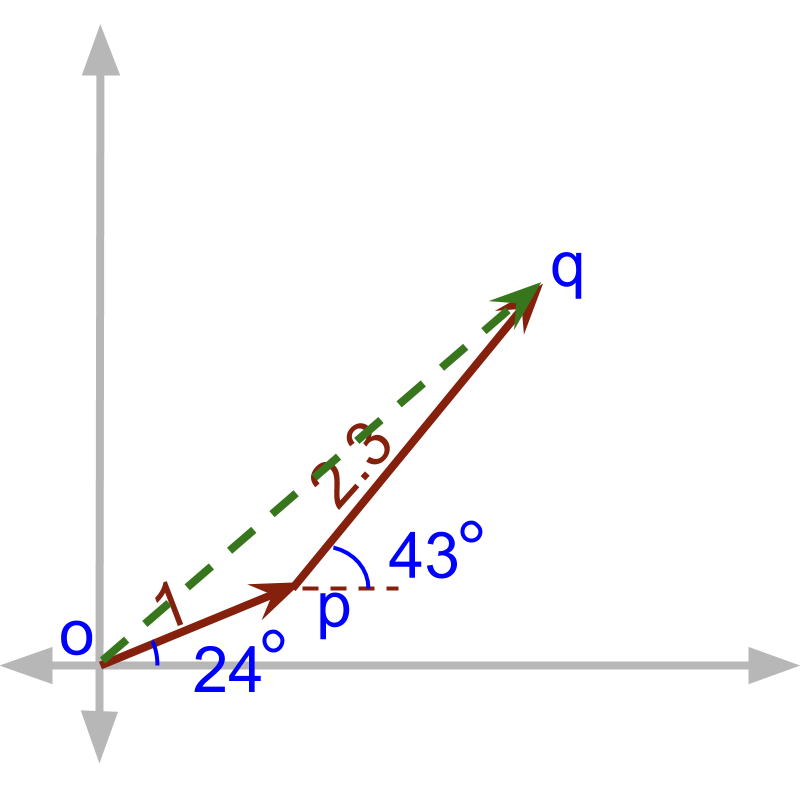maths > vector-algebra

Triangular Law

what you'll learn...

Overview

»  sequential addition of $\stackrel{\to }{p}$$\vec{p}$ and $\stackrel{\to }{q}$$\vec{q}$→  the third side of the triangle formed by $\stackrel{\to }{p}$$\vec{p}$ and $\stackrel{\to }{q}$$\vec{q}$

sequential

In a bucket there is $20$$20$ liter water and another $10$$10$ liter water is poured in. The bucket has $20+10=30$$20 + 10 = 30$ liter of water.

With $20$$20$ liter, another $10$$10$ liter is added. Scalar quantities can be added sequentially.

Two taps simultaneously fill a bucket. One fills at $20$$20$ liter per hour and another fills at $10$$10$ liter per hour. At the end of an hour, the bucket has $20+10=30$$20 + 10 = 30$ liter of water.

Two streams of water are added into the bucket. Scalar quantities can be added continuously.

We have seen that two ways scalar quantities can be added - Sequentially and Continuously.A person walks for $1$$1$ units at ${24}^{\circ }$${24}^{\circ}$ , then from that point, walks for $2.3$$2.3$ units at ${43}^{\circ }$${43}^{\circ}$ angle. Addition of these two vectors is an example of Sequential addition of vectors. At the end of the first vector, the second vector starts and added.Two vectors are added sequentially as given in the figure. The result of the addition is 'the third side of the triangle'.

Two vectors form a triangle with the sum as the third side of the triangle.

summary

Triangular Property of vector addition: : When two vectors are added, arrange the initial point of the second vector to the terminal point of the first vector. In that position, complete the triangle with the two vectors as the two sides. The third side, connecting initial point of the first vector and the terminal point of the second vector, is the sum of the two vectors.

Outline